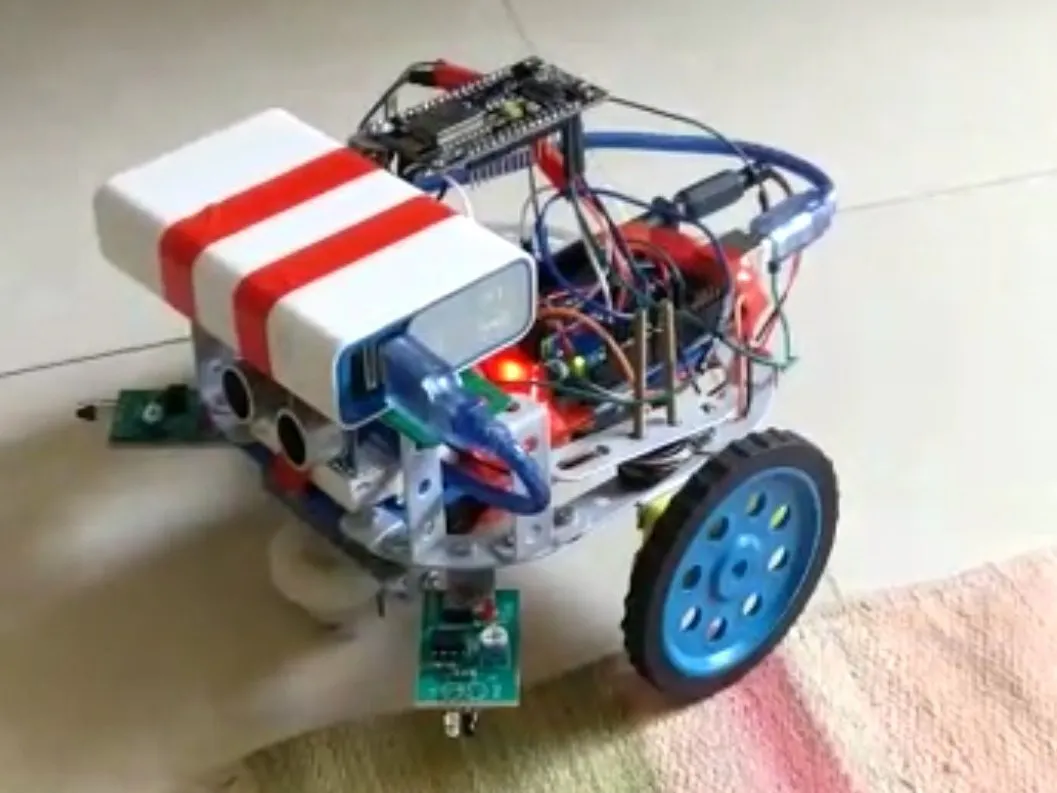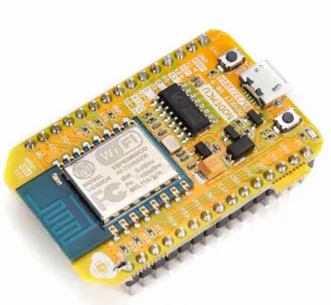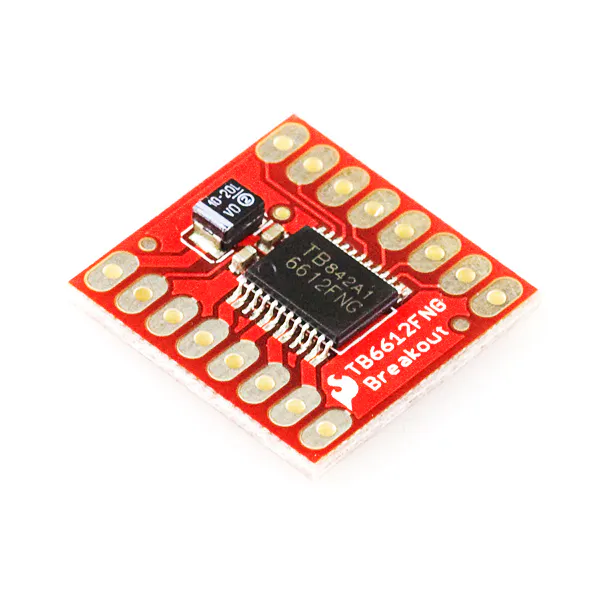# Remote Control bot

BLYNK app based remote used to control a bot.

IntermediateProtip1,951## Things used in this project

### Hardware componentsArduino UNO
×1NodeMCU ESP8266 Breakout Board
×1
 DC motor (generic)
×2SparkFun Motor Driver - Dual TB6612FNG (1A)
×1

### Software apps and online servicesBlynk

## Code

### Remote

C/C++
This code is for the wifi module
```#define BLYNK_PRINT Serial
#include <ESP8266WiFi.h>
#include <BlynkSimpleEsp8266.h>

// You should get Auth Token in the Blynk App.
// Go to the Project Settings (nut icon).
char auth[] = "6579f12f9e124f8f9fd0a3d2b3dae236";

// Set password to "" for open networks.
char ssid[] = "8085";
char pass[] = "boobnoob";
void setup(){
Blynk.begin(auth, ssid, pass);
pinMode(D0,OUTPUT);
pinMode(D1,OUTPUT);
pinMode(D2,OUTPUT);
pinMode(D3,OUTPUT);
Serial.println(9600);
}
void loop(){
Blynk.run();
}
```

### Arduino car code

C/C++
The code for driving the motors based on input.
```byte fw=2,bw=3,lt=4,rt=5;
byte la=8,lb=9,ra=10,rb=11;
byte f=0,r=0,l=0,b=0,state=0;
void setup() {
pinMode(fw,INPUT);
pinMode(bw,INPUT);
pinMode(lt,INPUT);
pinMode(rt,INPUT);
pinMode(lb,OUTPUT);
pinMode(la,OUTPUT);
pinMode(ra,OUTPUT);
pinMode(rb,OUTPUT);
Serial.begin(9600);
}
f=0;
r=0;
l=0;
b=0;
if(f==1)
{
Serial.println("out1");
return 10;
}
if(b==1)
{
Serial.println("out2");
return 20;
}
if(l==1)
{
Serial.println("out3");
return 30;
}
if(r==1)
{
Serial.println("out4");
return 40;
}
return 0;
}
void clearstate()
{
digitalWrite(ra,0);
digitalWrite(rb,0);
digitalWrite(la,0);
digitalWrite(lb,0);
}
void loop(){
while(state==10)
{
digitalWrite(la,1);
digitalWrite(lb,0);
digitalWrite(ra,1);
digitalWrite(rb,0);
}
clearstate();
while(state==20)
{
digitalWrite(la,0);
digitalWrite(lb,1);
digitalWrite(ra,0);
digitalWrite(rb,1);
}
clearstate();
while(state==30)
{
digitalWrite(la,0);
digitalWrite(lb,1);
digitalWrite(ra,1);
digitalWrite(rb,0);
}
clearstate();
while(state==40)
{
digitalWrite(la,1);
digitalWrite(lb,0);
digitalWrite(ra,0);
digitalWrite(rb,1);